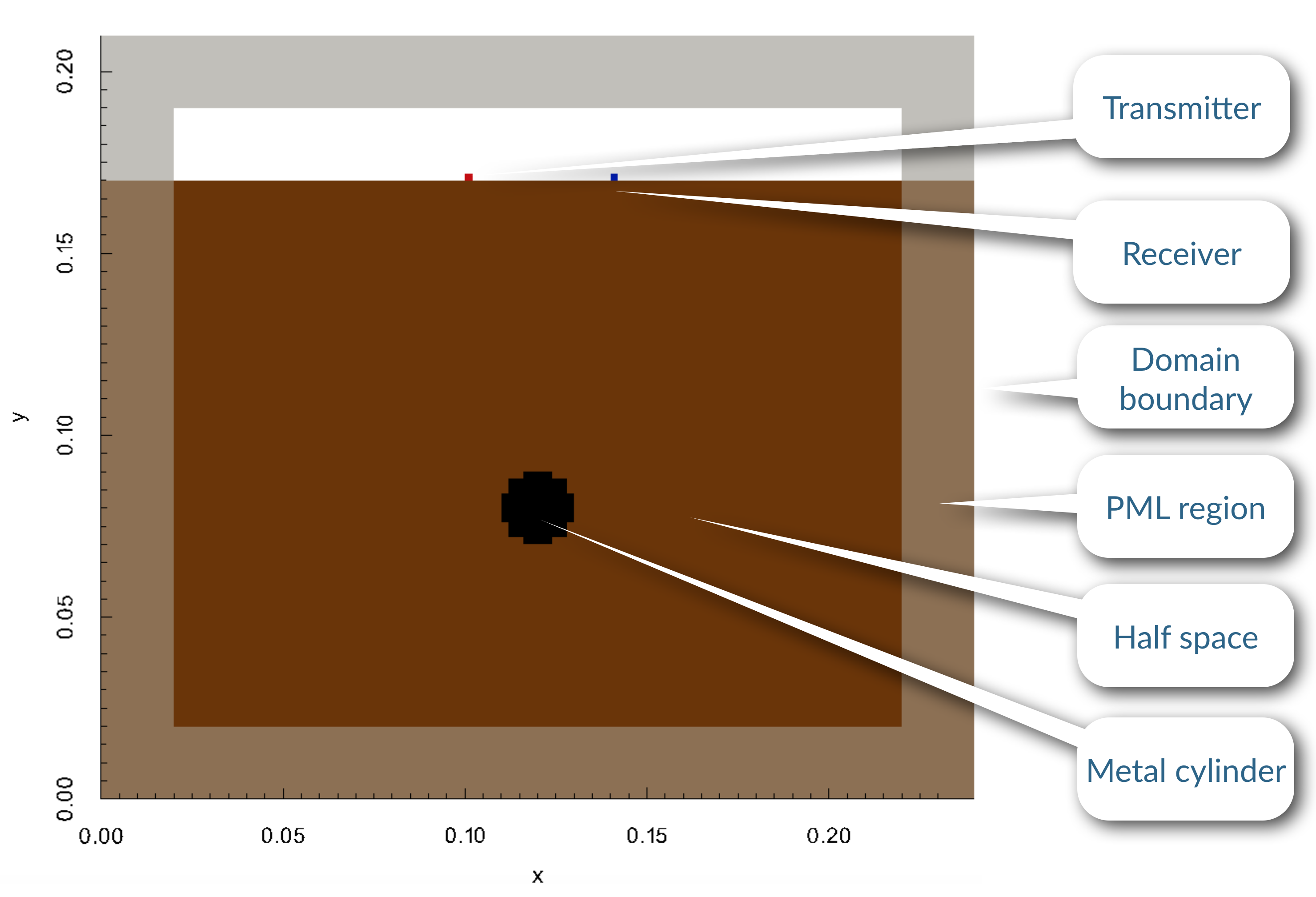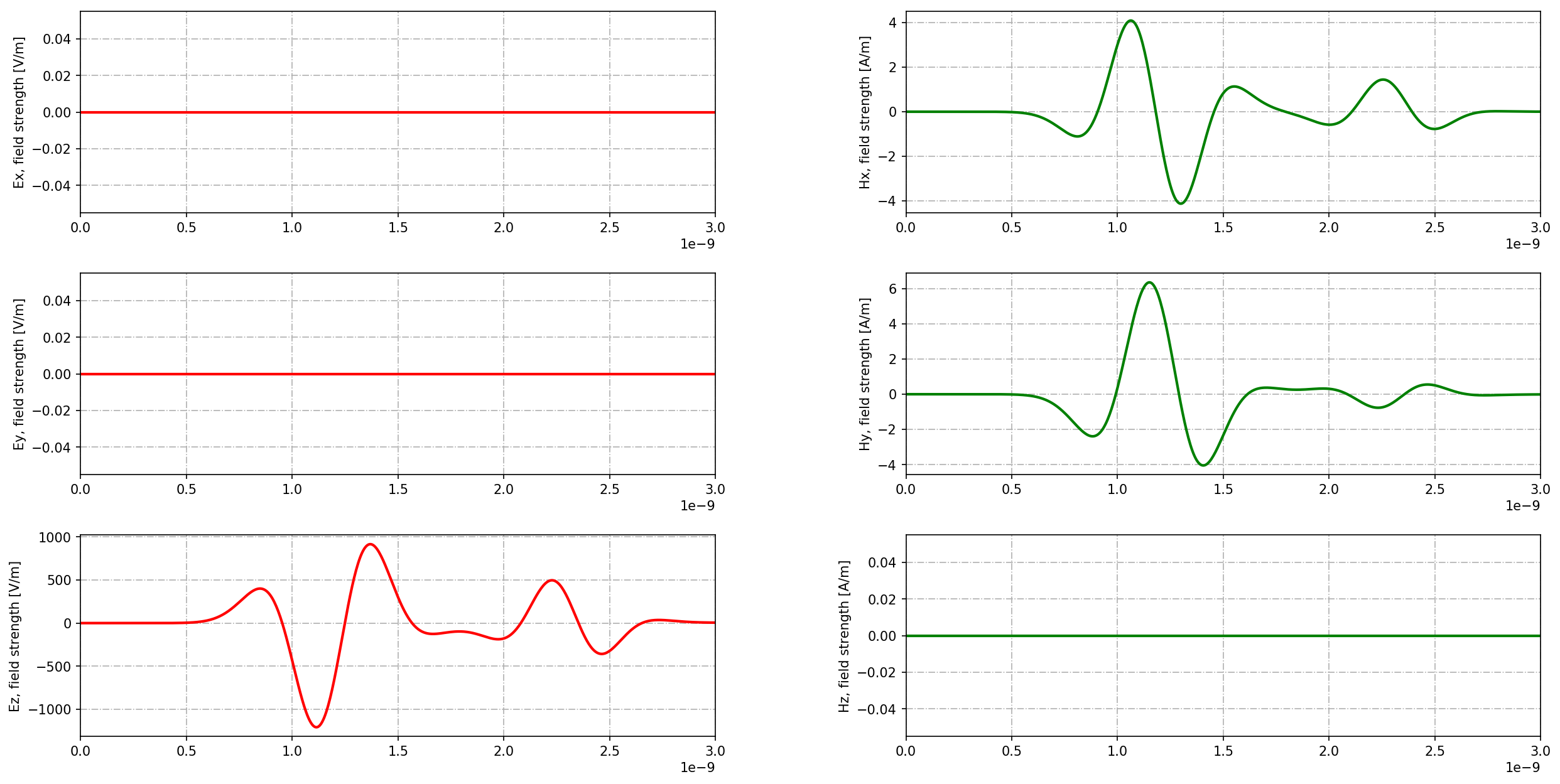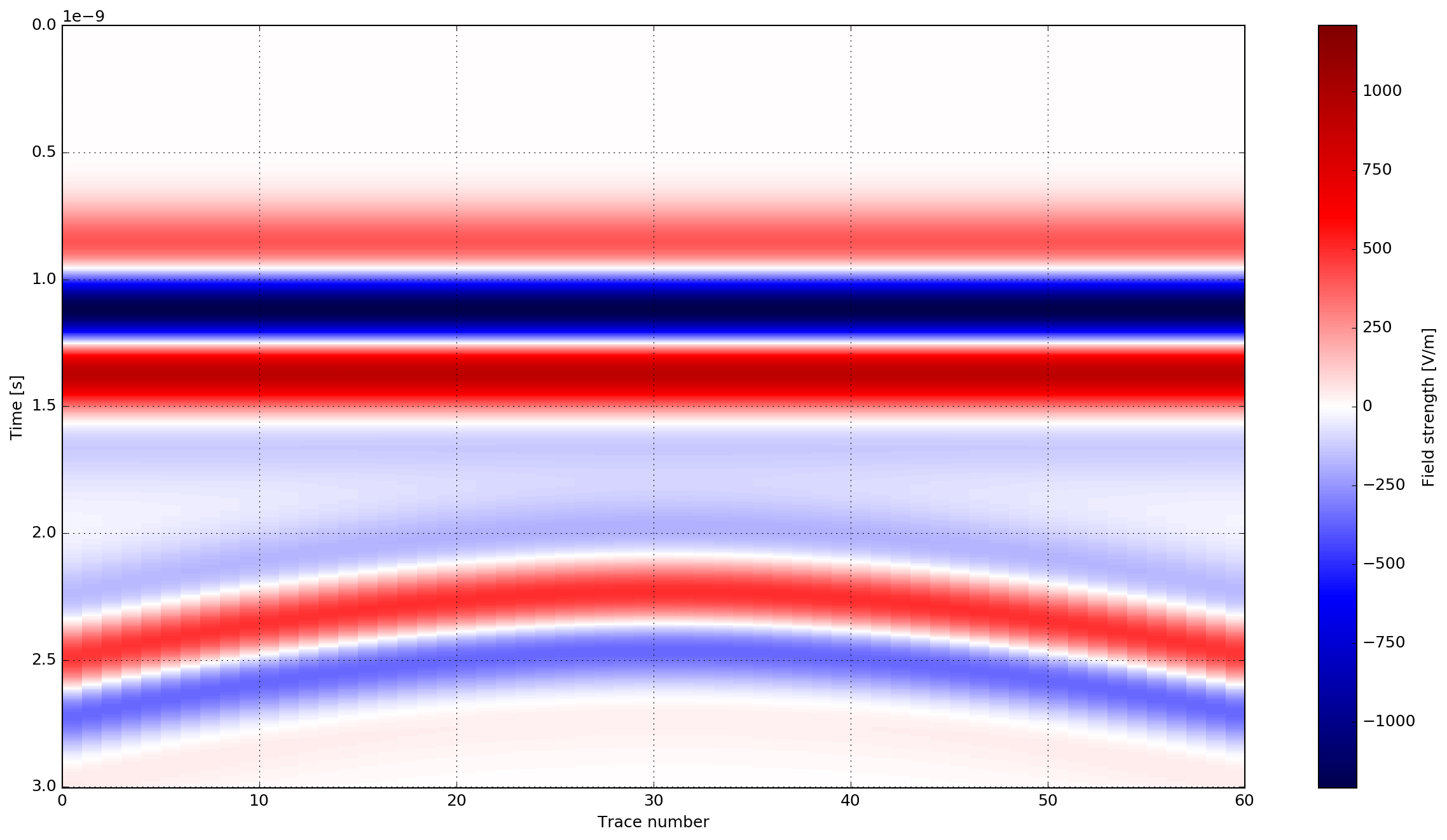# Introductory (2D) models¶

This section provides some introductory example models in 2D that demonstrate basic features of gprMax. Each example comes with an input file which you can download and run.

## A-scan from a metal cylinder¶

cylinder_Ascan_2D.in

This example is the GPR modelling equivalent of ‘Hello World’! It demonstrates how to simulate a single trace (A-scan) from a metal cylinder buried in a dielectric half-space.

  1 2 3 4 5 6 7 8 9 10 11 12 13 14 15 #title: A-scan from a metal cylinder buried in a dielectric half-space #domain: 0.240 0.210 0.002 #dx_dy_dz: 0.002 0.002 0.002 #time_window: 3e-9 #material: 6 0 1 0 half_space #waveform: ricker 1 1.5e9 my_ricker #hertzian_dipole: z 0.100 0.170 0 my_ricker #rx: 0.140 0.170 0 #box: 0 0 0 0.240 0.170 0.002 half_space #cylinder: 0.120 0.080 0 0.120 0.080 0.002 0.010 pec #geometry_view: 0 0 0 0.240 0.210 0.002 0.002 0.002 0.002 cylinder_half_space n 

The geometry of the scenario is straightforward and an image created from the geometry view is shown in Fig. 33. The transparent area around the boundary of the domain represents the PML region. The red cell is the source and the blue cell is the receiver.Fig. 33 Geometry of a 2D model of a metal cylinder buried in a dielectric half-space.

For this initial example a detailed description of what each command in the input file does and why each command was used is given. The following steps explain the process of building the input file:

### Determine the constitutive parameters for the materials¶

There will be three different materials in the model representing air, the dielectric half-space, and the metal cylinder. Air (free space) already exists as a built-in material in gprMax which can be accessed using the free_space identifier. The metal cylinder will be modelled as a Perfect Electric Conductor, which again exists as a built-in material in gprMax and can be accessed using the pec identifier. So the only material which has to be defined is for the dielectric half-space. It is a non-magnetic material, i.e. $$\mu_r=1$$ and $$\sigma_*=0$$ and with a relative permittivity of six, $$\epsilon_r=6$$, and zero conductivity, $$\sigma=0$$. The identifier half_space will be used.

#material: 6 0 1 0 half_space


### Determine the source type and excitation frequency¶

These should generally be known, often based on the GPR system or scenario being modelled. Low frequencies are used where significant penetration depth is important, whereas high frequencies are used where less penetration and better resolution are required. In this case a theoretical Hertzian dipole source fed with a Ricker waveform with a centre frequency of $$f_c=1.5~\textrm{GHz}$$ will be used to simulate the GPR antenna (see the bowtie antenna example model for how to include a model of the actual GPR antenna in the simulation).

#waveform: ricker 1 1.5e9 my_ricker
#hertzian_dipole: z 0.100 0.170 0 my_ricker


The Ricker waveform is created with the #waveform command, specifying an amplitude of one, centre frequency of 1.5 GHz and picking an arbitrary identifier of my_ricker. The Hertzian dipole source is created using the #hertzian_dipole command, specifying a z direction polarisation (perpendicular to the survey direction if a B-scan were being created), location on the surface of the slab, and using the Ricker waveform already created.

### Calculate a spatial resolution and domain size¶

In the Guidance on GPR modelling section it was stated that a good rule-of-thumb was that the spatial resolution should be one tenth of the smallest wavelength present in the model. To determine the smallest wavelength, the highest frequency and lowest velocity present in the model are required. The highest frequency is not the centre frequency of the Ricker waveform! By examining the spectrum of a Ricker waveform it is evident much higher frequencies are present, i.e. at a level -40dB from the centre frequency, frequencies 2-3 times as high are present. In this case the highest significant frequency present in the model is likely to be around 4 GHz. The wavelength at 4 GHz in the half-space (which has the lowest velocity) would be:

$\lambda = \frac{c}{f \sqrt{\epsilon_r}} = \frac{299792458}{4\times 10^9 \sqrt{6}} \approx 31~\textrm{mm}$

This would give a minimum spatial resolution of 3 mm. However, the diameter of the cylinder is 20 mm so would be resolved to 7 cells. Therefore a better choice would be 2 mm which resolves the diameter of the rebar to 10 cells.

#dx_dy_dz: 0.002 0.002 0.002


The domain size should be enough to enclose the volume of interest, plus allow 10 cells (if using the default value) for the PML absorbing boundary conditions and approximately another 10 cells of between the PML and any objects of interest. In this case the plan is to take a B-scan of the scenario (in the next example) so the domain should be large enough to do that. Although this is a 2D model one cell must be specified in the infinite direction (in this case the z direction) of the domain.

#domain: 0.240 0.210 0.002


### Choose a time window¶

It is desired to see the reflection from the cylinder, therefore the time window must be long enough to allow the electromagnetic waves to propagate from the source through the half-space to the cylinder and be reflected back to the receiver, i.e.

$t = \frac{0.180}{\frac{c}{\sqrt{6}}} \approx 1.5~\textrm{ns}$

This is the minimum time required, but the source waveform has a width of 1.2 ns, to allow for the entire source waveform to be reflected back to the receiver an initial time window of 3 ns will be tested.

#time_window: 3e-9


The time step required for the model is automatically calculated using the CFL condition in 2D.

### Create the objects¶

Now physical objects can be created for the half-space and the cylinder. First the #box command will be used to create the half-space and then the #cylinder command will be given which will overwrite the properties of the half-space with those of the cylinder at the location of the cylinder.

#box: 0 0 0 0.240 0.170 0.002 half_space
#cylinder: 0.120 0.080 0 0.120 0.080 0.002 0.010 pec


### Run the model¶

You can now run the model:

python -m gprMax user_models/cylinder_Ascan_2D.in


Tip

• You can use the --geometry-only command line argument to build a model and produce any geometry views but not run the simulation. This option is useful for checking the geometry of the model is correct.

### View the results¶

You should have produced an output file cylinder_Ascan_2D.out. You can view the results (see Output data section) using the command:

python -m tools.plot_Ascan user_models/cylinder_Ascan_2D.out


Fig. 34 shows the time history of the electric and magnetic field components and currents at the receiver location. The $$E_z$$ field component can be converted to voltage which represents the A-scan (trace). The initial part of the signal (~0.5-1.5 ns) represents the direct wave from transmitter to receiver. Then comes the reflected wavelet (~1.8-2.6 ns), which has opposite polarity, from the metal cylinder.Fig. 34 Electric and magnetic field component time histories from the receiver in the model of a metal cylinder buried in a dielectric half-space.

Check out a video of the field propagation in this example. More videos are screencasts can be found in the screencasts section.

## B-scan from a metal cylinder¶

cylinder_Bscan_2D.in

This example uses the same geometry as the previous example but this time a B-scan is created. A B-scan is composed of multiple traces (A-scans) recorded as the source and receiver are moved over the target, in this case the metal cylinder.

  1 2 3 4 5 6 7 8 9 10 11 12 13 14 15 #title: B-scan from a metal cylinder buried in a dielectric half-space #domain: 0.240 0.210 0.002 #dx_dy_dz: 0.002 0.002 0.002 #time_window: 3e-9 #material: 6 0 1 0 half_space #waveform: ricker 1 1.5e9 my_ricker #hertzian_dipole: z 0.040 0.170 0 my_ricker #rx: 0.080 0.170 0 #src_steps: 0.002 0 0 #rx_steps: 0.002 0 0 #box: 0 0 0 0.240 0.170 0.002 half_space #cylinder: 0.120 0.080 0 0.120 0.080 0.002 0.010 pec 

The differences between this input file and the one from the A-scan are the x coordinates of the source and receiver (lines 11 and 12), and the commands needed to move the source and receiver (lines 13 and 14). As before, the source and receiver are offset by 40mm from each other as before but they are now shifted to a starting position for the scan. The #src_steps command is used to move every source in the model by specified steps each time the model is run. Similarly, the #rx_steps command is used to move every receiver in the model by specified steps each time the model is run. Note, the same functionality can be achieved by using a block of Python code in the input file to move the source and receiver individually (for further details see the Python section).

To run the model to create a B-scan you must pass an optional argument to specify the number of times the model should be run. In this case this is the number of A-scans (traces) that will comprise the B-scan. For a B-scan over a distance of 120mm with a step of 2mm that is 60 A-scans.

python -m gprMax user_models/cylinder_Bscan_2D.in -n 60


### Results¶

You should have produced 60 output files, one for each A-scan, with names cylinder_Bscan_2D1.out, cylinder_Bscan_2D2.out etc… These can be combined into a single file using the command:

python -m tools.outputfiles_merge user_models/cylinder_Bscan_2D


You should see a combined output file cylinder_Bscan_2D_merged.out. You can add the optional argument --remove-files if you want to automatically delete the original single A-scan output files.

You can now view an image of the B-scan using the command:

python -m tools.plot_Bscan user_models/cylinder_Bscan_2D_merged.out Ez


Fig. 35 shows the B-scan (of the $$E_z$$ field component). Again, the initial part of the signal (~0.5-1.5 ns) represents the direct wave from transmitter to receiver. Then comes the refelected wave (~2-3 ns) from the metal cylinder which creates the hyperbolic shape.Fig. 35 B-scan of model of a metal cylinder buried in a dielectric half-space.